# Particle, Antiparticle & Photons

## Introduction

Every particle has an antiparticle, according to the standard model for describing fundamental particles and interactions. For example, the positron is the antiparticle of the electron. A positron has identical mass, but has a positive charge. If an electron encounters a positron, they annihilate with the transformation of their mass-energies into two gamma rays. In this article, the properties of particles and their antiparticles are explained.

A. Antiparticles

Antiparticle can be defined as a subatomic particle having the same mass as a given particle but opposite electric or magnetic properties. Every subatomic particle has a corresponding antiparticle. Figure 1 shows some of the particles and their antiparticles.

Figure 1: Particle and Antiparticle

B. Electron & Positron

A positron, also called a positive electron, is a positively-charged subatomic particle having the same mass and magnitude of charge as the electron. Properties of the electron and positron are presented in the following table:C. Proton & Antiproton

An antiproton is a subatomic particle. It is the proton’s antiparticle. Antiprotons have the same mass as a proton, but have a negative electric charge and oppositely directed magnetic moment. Properties of a proton and antiproton are presented in the following table:D. Neutron & Antineutron

An antineutron is a subatomic particle. It is a neutron’s antiparticle. It has the same mass as the neutron, and no net electric charge, but has an opposite Baryon number (+1 for neutron, −1 for the antineutron). This is because the antineutron is composed of antiquarks, while neutrons are composed of quarks. The properties of a neutron and antineutron are presented in the following table:E. Neutrino and Antineutrino

An antineutrino is a subatomic particle. For each neutrino, there also exists a corresponding antiparticle, called an antineutrino. Like the neutrino, an antineutrino also has no electric charge and half-integer spin. They are distinguished from neutrinos by having opposite signs of the Lepton number. The properties of neutrinos and antineutrinos are presented in the following table: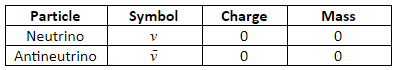F. Photon

According to a theory developed by Albert Einstein, light radiation consists of a stream of energy packets called photons. A photon is the special name given to a quantum of energy when the energy is in the form of electromagnetic radiation. The energy (E) carried in each quantum is given by the following equation: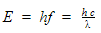Where, f – frequency of the radiation (Hz)

h – Plank constant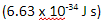c – Speed of light in vacuumλ – Wavelength (m).

G. Pair production

In pair production, a photon creates an electron and a positron. In the pair production process, the photon disappears. A positron has the same mass as an electron but has a positive charge. Since a photon has no mass, it is considered that pair production is an example of creating matter from pure energy. This is explained by the energy-mass equivalence, E = m c2. Pair production process is illustrated in Figure 2.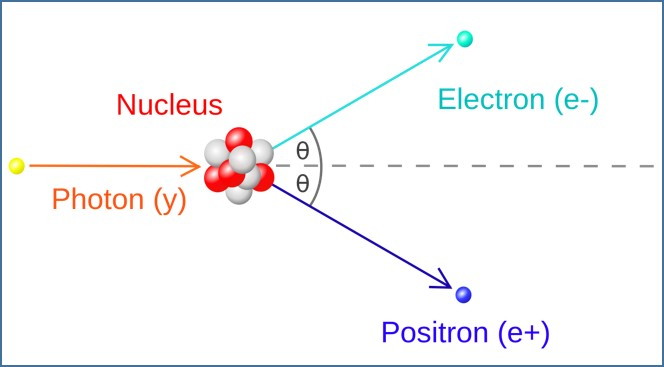Figure 2: Pair Production

H. Annihilation

Annihilation is a reaction in which a particle and its antiparticle collide and disappear, releasing energy. For example, an annihilation reaction occurs between an electron and its antiparticle, a positron. A positron that originates in radioactive decay usually combines briefly with an electron to form a quasi-atom called a positronium. The quasi-atom is composed of the two particles spinning around each other before they annihilate. After the annihilation, two or three gamma rays radiate from the point of collision. Annihilation is illustrated in Figure 3.Figure 3: Annihilation

The amount of energy (E) produced by annihilation is equal to the mass (m) that disappears multiplied by the square of the speed of light in a vacuum (c). The amount of energy (E) produced by annihilation is governed by the equation,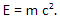I. Summary

• Antiparticles can be defined as subatomic particles having the same mass as a given particle but opposite electric or magnetic properties.
• Positrons, also called positive electrons, are positively charged subatomic particles having the same mass and magnitude of charge as electrons.
• Antiprotons are subatomic particles that have the same mass as a proton but a negative electric charge and oppositely directed magnetic moment.
• An antineutron is a subatomic particle that has the same mass as the neutron, and no net electric charge, but has an opposite Baryon number.
• An antineutrino is an antiparticle of a neutrino that has no electric charge and half-integer spin.
• A photon is a quantum of energy when the energy is in the form of electromagnetic radiation.
• In pair production, a photon creates an electron and positron. In the pair production process, the photon disappears.
• Annihilation is a reaction in which a particle and its antiparticle collide and disappear, releasing energy.